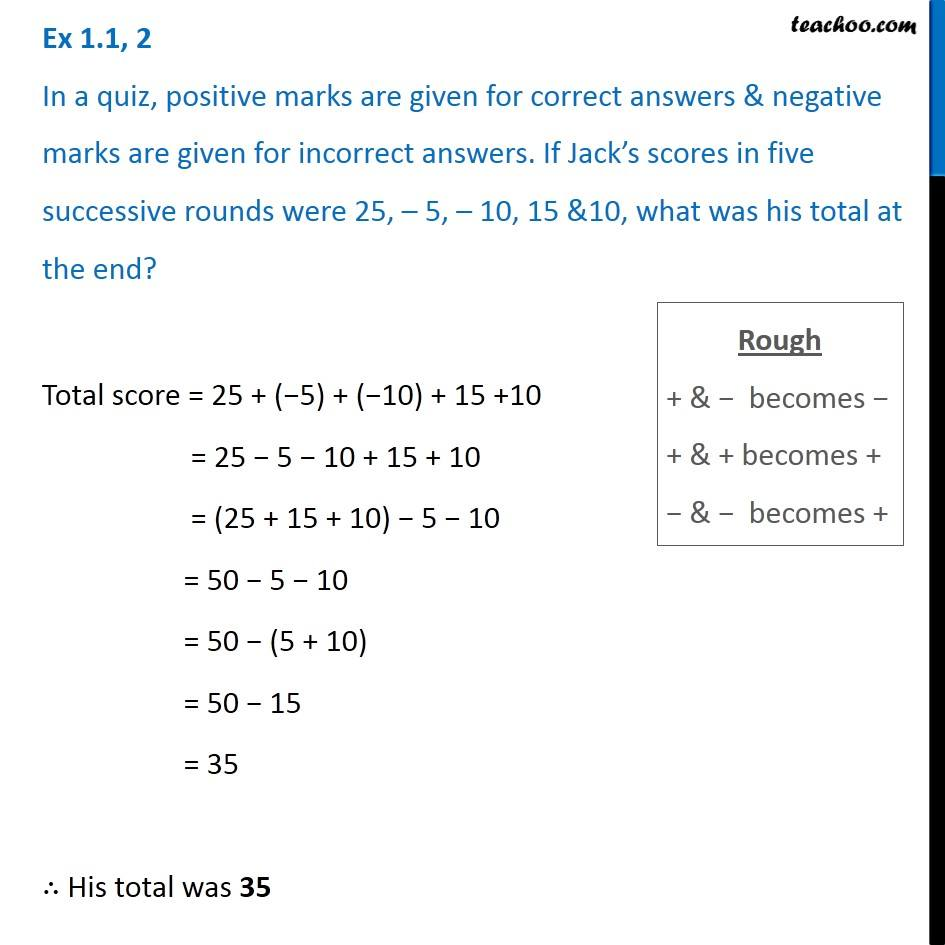Chapter 1 Class 7 Integers
Serial order wiseLearn in your speed, with individual attention - Teachoo Maths 1-on-1 Class

### Transcript

Question 2 In a quiz, positive marks are given for correct answers & negative marks are given for incorrect answers. If Jack s scores in five successive rounds were 25, 5, 10, 15 &10, what was his total at the end? Rough + & becomes + & + becomes + & becomes + Total score = 25 + ( 5) + ( 10) + 15 +10 = 25 5 10 + 15 + 10 = (25 + 15 + 10) 5 10 = 50 5 10 = 50 (5 + 10) = 50 15 = 35 His total was 35